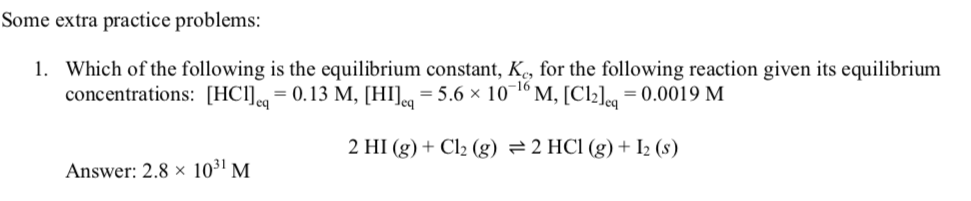# Which of the following is the equilibrium constant, Kc, for the following reaction given its equilibrium concentrations: [HCl]eq= 0.13 M, [HI]eq= 5.6 × 10^−16 M, [Cl2]eq= 0.0019 M2 HI (g)+ Cl2(g)⇌2HCl(g)+ I2(s) Answer: 2.8 × 10^31 M I keep getting the answer: 1.59 x 10^32